HOME / Applications / AC Transformer

# AC Transformer

Used Tools:## AC transformer

An AC transformer is an electrical device that is used to change the voltage in alternating current (AC) electrical circuits.
One of the great advantages of AC over DC for electric-power distribution is that it is much easier to step voltage levels up and down with AC than with DC. For long-distance power transmission it is desirable to use as high a voltage and as small a current as possible; this reduces R*I2 losses in the transmission lines, and smaller wires can be used, saving on material costs.

## Solid works model of an AC transformer

The Solid works model of an AC transformer consists of a C-Core, two inner coils and two outer coils, as shown in Figure 1.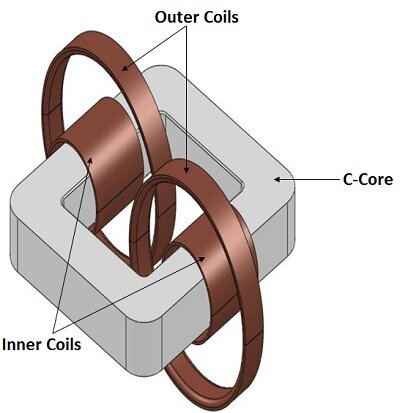Figure 1 - 3D model of the AC transformer

## EMS Simulation of an AC Transformer

In EMS, an AC transformer is analyzed using AC Magnetic study to calculate the core loss, the electromagnetic results.
Within this simulation, the frequency of the AC Magnetic study was set to be 50 Hz.

## Material

The simulated model composed of a Core, two inner coils, two outer coils, an inner air (around coils) and an outer coil. The properties of the materials are summarized in Table 1.

Table 1 - Materials used in the EMS simulation

 Component Material Relative permeability Electrical Conductivity (S/m) C-Core Steel 1 5000 1.030e+007 Inner Coils/ Outer Coils Copper 0.99991 5.7e+007 Inner Air, Outer Air Air 1 0

## Coils

In this simulation, the two inner and the two outer coils are modeled as wound coils. They operate at 50 Hz.
Figure 2 illustrates the current entry and exit of the inner and outer coils.
Coils properties (Number of turns and current) are presented in Table 2.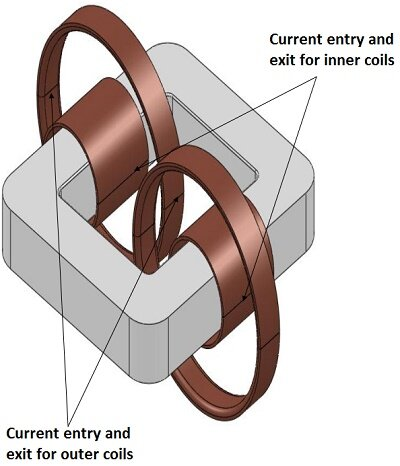Figure 2 - Current entry and exit for inner and outer coils

Table 2 - Coils information

 Name Number of turns Current Magnitude (RMS) Current Phase Inner Coils (primary) 21 15.5 A 0 deg Outer Coils (Secondary) 22 15.5 A 0 deg

## Meshing

Meshing is a very crucial step in the design analysis. EMS estimates a global element size for the model taking into consideration its volume, surface area, and other geometric details. The quality of mesh plays a key role in the accuracy of the results.

Mesh control refers to specifying different element sizes at different regions in the model. A smaller element size in a region improves the accuracy of results in that region.

Figure 3 is the meshed model after using Mesh Controls (Table 2).

Table 2 - Mesh control

 Name Mesh size Components /Bodies Mesh control 1 10.00 mm C-Core Mesh control 2 5.00 mm Inner Coils Mesh control 3 7.00 mm Outer Coils Mesh control 4 20.00 mm Inner Air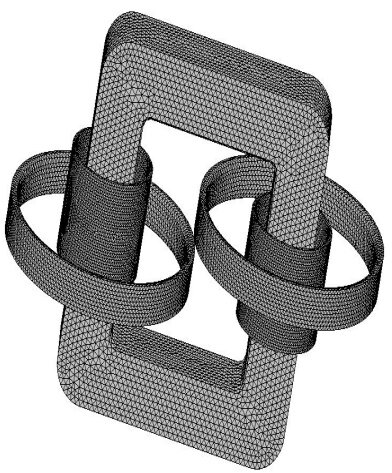Figure 3 - Meshed model

## Results

In AC Magnetic study, EMS computes magnetic flux density, magnetic field intensity, force density, electric field, inductances, resistances, flux linkage, leakage inductance, eddy current, induced voltage, energy and losses.

The computed Ohmic loss, Eddy loss, Hysteresis loss, Excess loss and Core loss in the C-Core body are shown in Figure 4.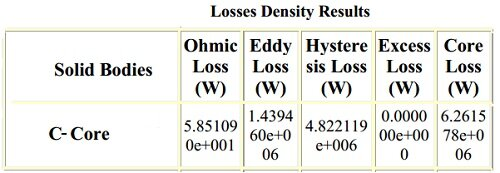Figure 4 - Losses density results

## 3D fields generated by EMS

The 3D plot of the magnetic flux density, the current density and the Core loss in the transformer generated by EMS, are shown in Figures 5, 6 and 7 respectively.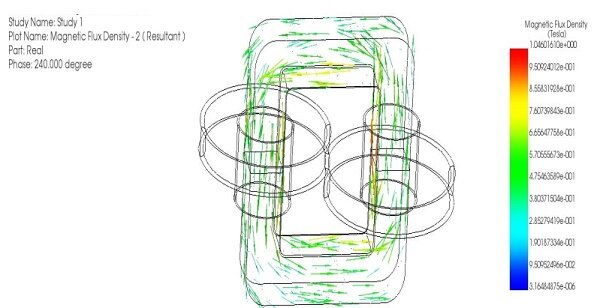Figure 5 - Magnetic flux density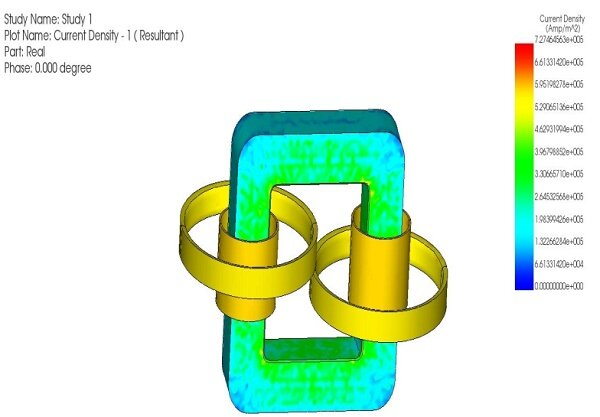Figure 6 - Current density distribution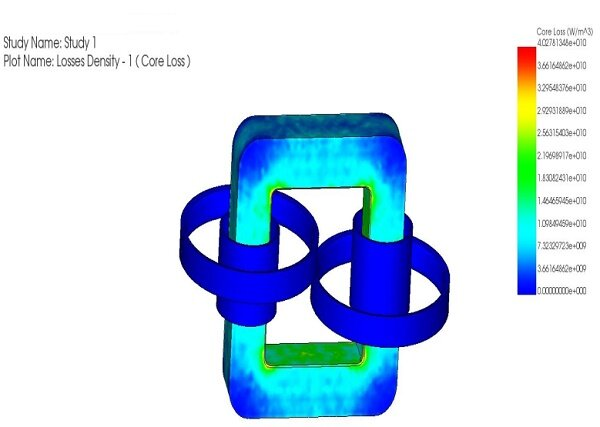Figure 7 - Core loss in the transformer

## Conclusion

This example shows that using EMS, computation of the AC transformers parameters, such as the core loss, the magnetic flux density, the current density, the Ohmic loss, the electric field, the force density and other electromagnetic parameters, is easily performed.

Upcoming Webinars